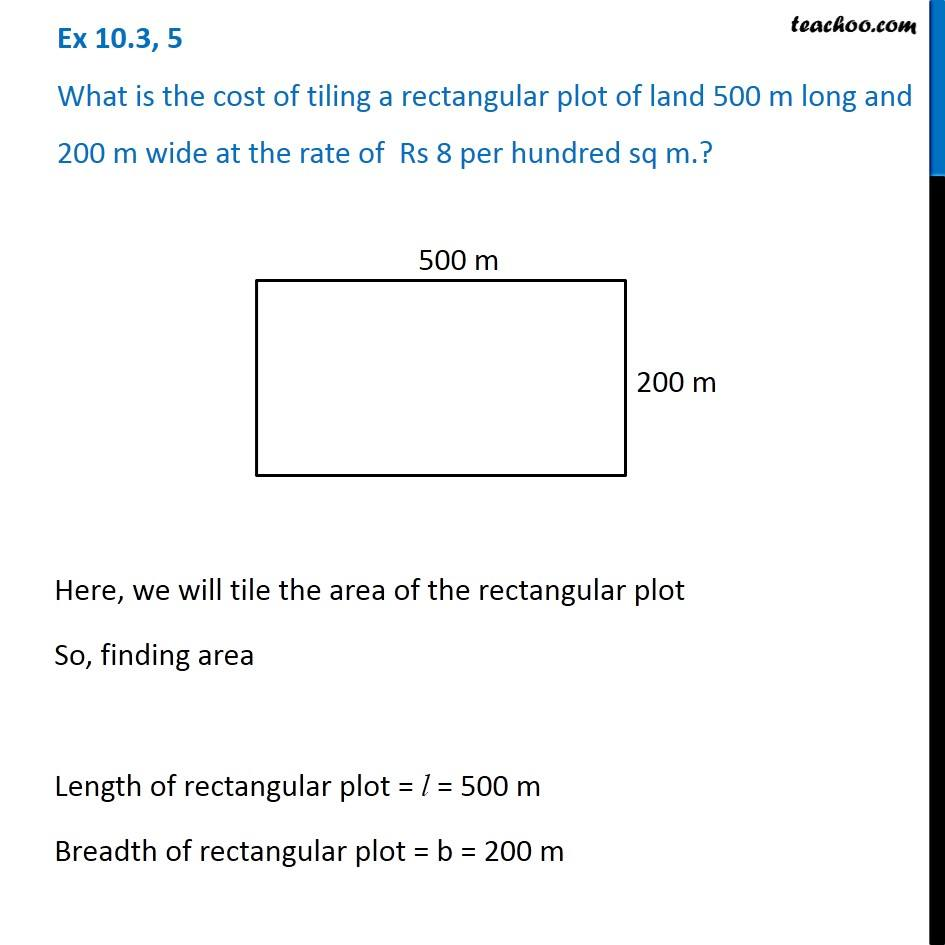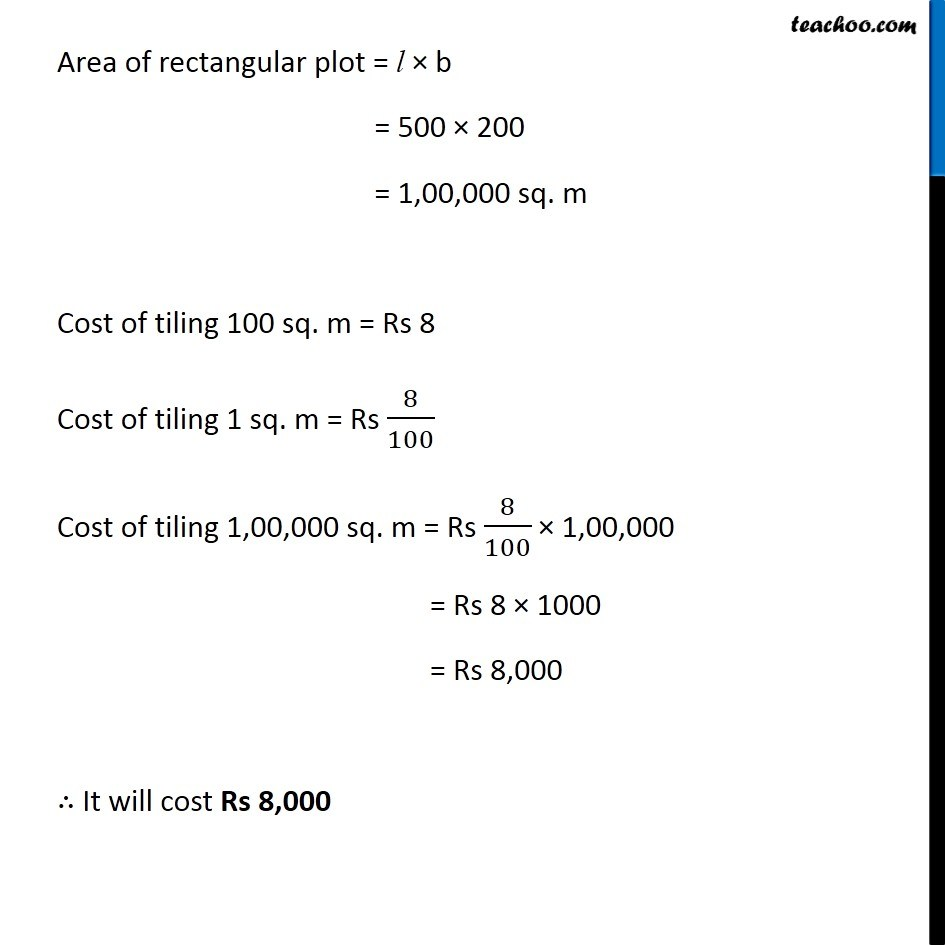1. Chapter 10 Class 6 Mensuration
2. Concept wise
3. Area of Rectangle

Transcript

Ex 10.3, 5 What is the cost of tiling a rectangular plot of land 500 m long and 200 m wide at the rate of Rs 8 per hundred sq m.? Here, we will tile the area of the rectangular plot So, finding area Length of rectangular plot = l = 500 m Breadth of rectangular plot = b = 200 m Area of rectangular plot = l × b = 500 × 200 = 1,00,000 sq. m Cost of tiling 100 sq. m = Rs 8 Cost of tiling 1 sq. m = Rs 8/100 Cost of tiling 1,00,000 sq. m = Rs 8/100 × 1,00,000 = Rs 8 × 1000 = Rs 8,000 ∴ It will cost Rs 8,000

Area of Rectangle

About the AuthorDavneet Singh
Davneet Singh is a graduate from Indian Institute of Technology, Kanpur. He has been teaching from the past 9 years. He provides courses for Maths and Science at Teachoo.